Courses

# Process Costing - Methods of Costing, Cost Accounting B Com Notes | EduRev

## B Com : Process Costing - Methods of Costing, Cost Accounting B Com Notes | EduRev

The document Process Costing - Methods of Costing, Cost Accounting B Com Notes | EduRev is a part of the B Com Course Cost Accounting.
All you need of B Com at this link: B Com

Process Costing
Process costing is the method of costing applied in the industries engaged in continuous or mass production. Process costing is a method of costing used to ascertain the cost of a product at each process or stage of manufacturing.
According to ICMA terminology, “Process Costing is that form of operation costing which applies where standardized goods are produced”.
So it is a basic method to ascertain the cost at each stage of manufacturing. Separate accounts are maintained at each process to which expenditure incurred. At the end of each process the cost per unit is determined by dividing the total cost by the number of units produced at each stage. Hence, this costing is also called as “Average Costing” or “Continuous Costing”. Process Costing is used in the industries like manufacturing industries, chemical industries, mining works and public utility undertakings.

Characteristics of Process Costing

1. Production is continuous
2. Products pass through two or more distinct processes of completion.
3. Products are standardized and homogeneous.
4. Products are not distinguishable in processing stage.
5. The finished product of one process becomes the raw material of the subsequent process.
6. Cost of material, labour and overheads are collected for each process and charged accordingly.

1. It is easy to compute average cot because the products are homogeneous in Process Costing.
2. It is possible to ascertain the process costs at short intervals.
3. Process Costing is simple and less expensive in relation o job costing.
4. By evaluating the performance of each process effective managerial control is possible.

1. Valuation of work in progress is difficult.
2. It is not easy to value losses, wastes, scraps etc.
3. The apportionment of total cost among joint products and by-products is difficult.
4. Process cost are not accurate, they are only average costs 5. Process costs are only historical.

Principles of Process Costing
The following points are considered while determining the cost under Process Costing.

1. Production activity should be divided into different processes or departments.
2. A separate account is opened for each process.
3. Both direct and indirect costs are collected for each process.
4. The quantity of output and costs are recorded in the respective process accounts.
5. The cost per unit is determined by dividing the total cost at the end of each process by the number of output of each process.
6. Normal loss and abnormal loss are credited in the process account
7. The accumulated cost of each process is transferred to subsequent process along with output. The output of the last process along with cost is transferred to the finished goods account.
8. In case of by-products and joint products their share in joint cost should be estimated and credited to the min process.
9. When there is work in progress at the end of the period the computation of inventory is made I terms of complete units

Difference between Process Costing and Job Costing

 Process Costing Job Costing 1. Production is continuous 1.Production is according to customers’ orders 2. Production is for stock 2. Production is not for stock 3. All units produced are identical or homogeneous 3. Each job is different from the other 4. There is regular transfer of cost of one process to subsequent processes 4. There is no regular transfer of cost from one job to another 5. Work in progress always exists 5. Work in progress may or may not exist

Procedure for Process Costing

1. Each process is separately identified. Separate process account is opened for each process.
2. Along with ‘Particulars Column’, two columns are provided on both sides of the process account – units (quantity) and amount (Rupees).
3. All the expenses are debited in the respective process account.
4. Wastage, sale of scrap, by-products etc are reordered on the credit side 0f the process account.
5. The difference between debit and credit side shows the cost of production and output of that particular process which is transferred to the next process.
6. The cost per unit in every process is calculated by dividing the net cost by the output.
7. The output of last process is transferred to the Finished Stock Account.
8. Incomplete units at the end of the each period ion every process s converted in terms of completed units

TYPES OF PROCESS COSTING
There are three types of process costing which can be used in different situations.

1. Weighted Average Costs – This is done by dividing the cost of goods that are available for sale by the number of products that are available for sale which will give you an average cost per unit.
2. Standard Costs – This is similar to the weighted average method but uses a standard cost rather than the actual costs. Once this is measured, they are then matched against the actual costs incurred and are the difference is charged to another variance account. This is used when a company produces in large batches but have a varied mix of products to which they cannot assign separate costs to.
3. First In First Out (FIFO) – This is the method of valuation where the first goods purchased are the first ones sold which is one of the best methods for a business to use as the risk of inventory going obsolete is reduced. It is a very complex calculation that uses layers of costs to account for significant changes in costs over time. This method is the most logically correct of all the process costing methods, and similarly, it is the hardest while the Weighted Average method is the easiest.

THE 5 STEPS FOR PROCESS COSTING
Now that we have a basic idea of what process costing is let’s move on to the full method of how process is costing works.
There are basically five steps in this method that a business would have to go through:

1. Analyze the flow of actual units
2. Convert the inventory to determine the equivalent units
3. Identify the total costs
4. Calculate the average cost per equivalent unit
5. Allocate these costs to finished units and Work in Process units

1. Analyze the Flow of Actual Units
The first thing that the business should do is to identify the flow of units during the manufacturing process.
This type of costing relies on this distinct flow of units as it will determine exactly how the costs should be added on during the whole process.
As you read about before, when producing homogenous products there are usually several production processes involved.
Once these processes are identified, the costs will be added to each process in sequence until you get a final value that determines the cost related to that specific method of production.
Different manufacturers will have varying types of processes which can range from just two to over a dozen which will change the way costing is done drastically from each type of business.

2. Convert the Inventory to Determine the Equivalent Units
Equivalent units are the number of units that are completed during a certain stage of production.
As the production moves along the line, it is inevitable that not all units will be completed from one stage to the next.
The units that are not fully complete when it moves on to the next process are called “Work in Process”.
These units are calculated based on their stage of completion and how many processes it has gone through until now.
This percentage will depend on the type of business and the value that they set on which process the unit should be in to be called an equivalent unit.
For example, a furniture manufacturer may identify a unit as “complete” when it passes the cutting or assembling processes but are yet to go through the polishing process.
Whereas, a wine manufacturer would not be able to call their units complete until they have passed the aging and bottling stage which would take a long time in the production process.
So if production during that stage has 4 processes and 3 are completed, then the unit would be 75% complete which could be counted as an equivalent unit for the company.
Taking all this into account, the company should then determine the total number of equivalent units produced during that period by taking the total number of Work in Process units and multiplying it with the units that are complete. (Ex. 5000 Work in Process units x 0.75 {75%} = 3750 completed units).
If you are looking to know more about process costing, here is a video that talks about it in a simpler way.

3. Identify the Total Costs
The third step is to account for all the costs that are incurred during the whole production process.
This is done by adding costs to each process to get an average individual cost per unit.
Compared to the other costing methods available, this method uses quite a basic method to calculate these costs.
These costs are divided into direct costs and indirect costs.
The direct costs are usually noted down as:

• Direct Labor – This is the cost of employing people to work on producing the product during that particular process. Ex: Factory worker on the bottling line.
• Direct Materials – These are the costs of the raw materials that were used to produce the units during the process.
• Manufacturing Overheads – This includes all the other costs that will be incurred during this time that are not accounted for in Direct Costs such as depreciation, rent, property tax, and electricity.

Other examples of indirect costs would include facility maintenance, worker salaries, quality assurance, and other factory supplies which are not directly related to manufacturing.

4. Calculate the Average Cost Per Equivalent Unit
Once all the costs have been identified for each process, then it’s a simple process to calculate the average cost per unit.
All of these costs from each process are added together to get one total cost, and this value is then divided by the total equivalent unit number to get the average equivalent cost per unit.

Example
Soda Manufacturing
Soda Production (First Process) – DM (\$100,000), CC (\$200,000)
Bottling (Second Process) – DM (\$40,000), CC (\$50,000)
Labeling (Third Process) – DM (\$25,000), CC (\$25,000)
Sealing (Fourth Process) – DM (\$10,000), CC (\$10,000)
Work in Process units – 100,000 units x 0.25 (Assuming completed percentage is 75%) = 25000 units
Total Costs – DM (\$175,000) + CC (\$285,000) = \$460,000
Average Cost per Unit = \$460,000/75000 units = \$6.13 per unit

5. Allocate Costs to Finished Units and Work in Process Units
If you are looking to get the most accurate value of the costs incurred, here is the thing.
It’s not just the value of the equivalent costs that must be taken into account for that period.
Even if the Work in Process is half finished, they still incurred a cost during that period which must be added on as well.
Each stage of production will always have an opening inventory and closing inventory value which includes the Work in Process units that were brought forward from the last period of production.
To show this in an example, let’s say that 10,000 units which were halfway complete from the previous production period were brought forward as opening inventory.

Soda Manufacturing
Inventory brought forward – 5000 units (10,000 units * 0.50 {completion percentage from previous period})
Opening Inventory Value = 5000 units * \$6 per unit (Value to produce these units since last period) = \$30,000
Current Work in Process Units – 12500 units (25,000 units * 0.50 {completion percentage from current period})
Total Average Cost for the period = 75,000 units + 12,500 units = 87,500 units
\$460,000 + \$30,000 (Opening Inventory Value) = \$490,000
\$490,000/87,500 units = \$5.6 per unit
Rather than calculating the cost based only on the equivalent units, the business can get a much more accurate value by including the Work in Process units too as they were also involved in the current period of production and so consumed direct material, direct labour, and other manufacturing costs as well.
It is also the same with the opening stock from the previous period as they were only halfway complete but then fully completed in the current period but not up to the full extent.
By adding a cost to both the equivalent and work in process units, the company can make sure that they are not leaving any of the costs unturned and will give a more accurate view of exactly how much the units from that period would cost them.
As can be seen in the example, the cost reduced from \$6.13 per unit to \$5.6 with the inclusion of the work in process units which can be quite a large amount when dealing in mass production of products.

Process Costing Problems and Solution
Problem 1:
The Zee manufacturing company produces a single product which goes through one process only. The manufacturing cycle takes a month. The company started its manufacturing operation on 1st February, 2018 and costs of production for this month were as follows:
Material  Rs. 41,412;
Labor  Rs. 32,054;
FOH  Rs. 23,870
The production statistics for the month were as follow:
Units completed and transfer to finished goods store Rs. 6,500
Unit in Process on February 31, 2018 was 1,600 (Material 40%, Labor and FOH 20 per cent)
Requirement: Prepare the Cost of Production Report at the end of February, 2018
Solution: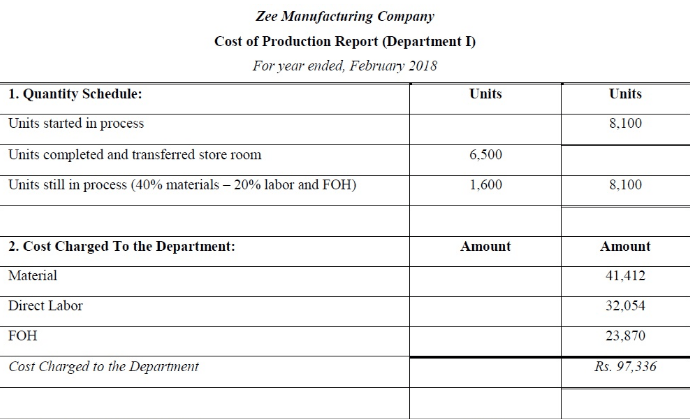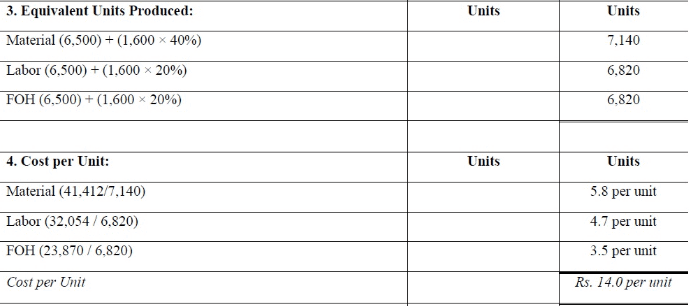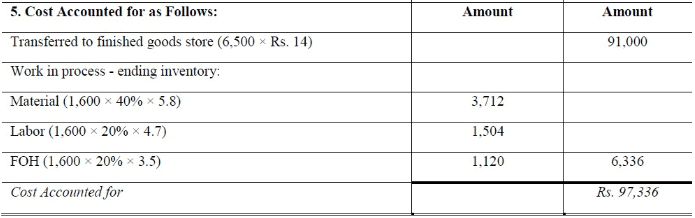Problem 2:
The Prince Corporation has two production departments and maintains a process cost system. The following is a summary of the cots for the month of December, 2017: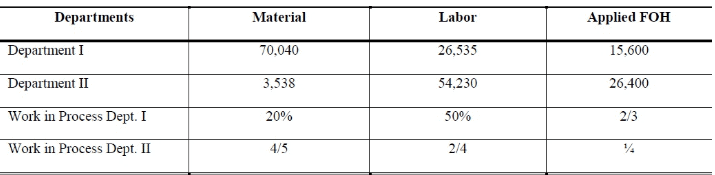Report form production control department shows as follows: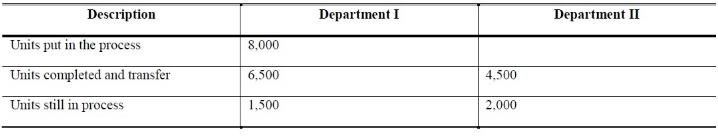Solution: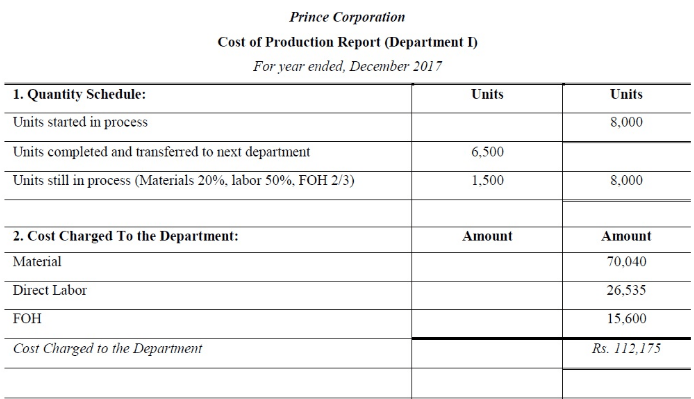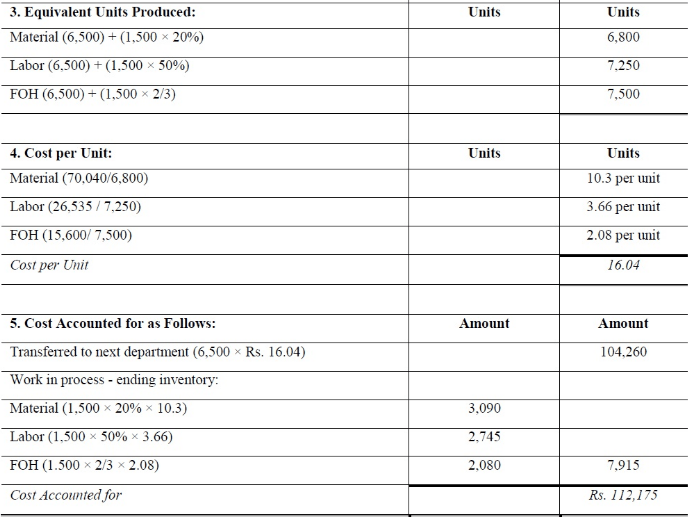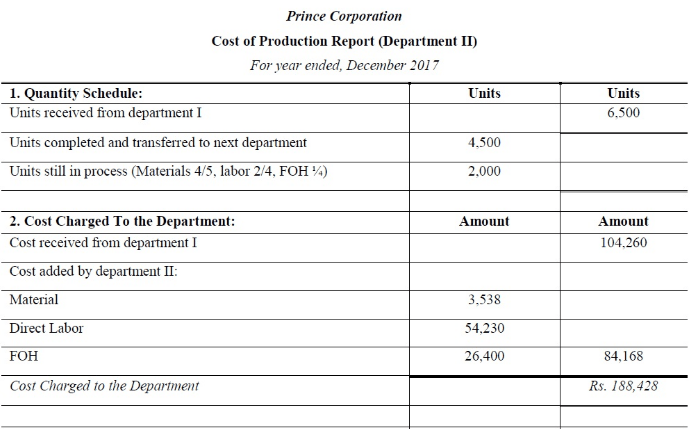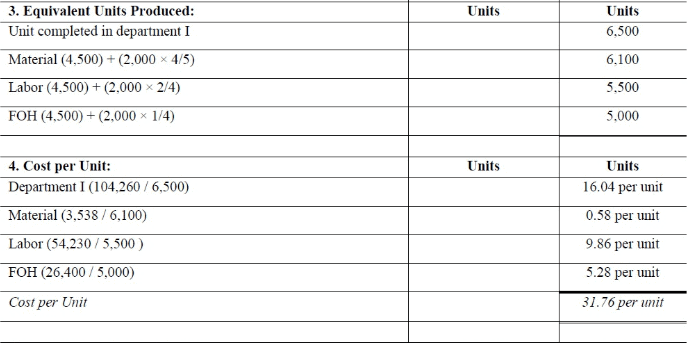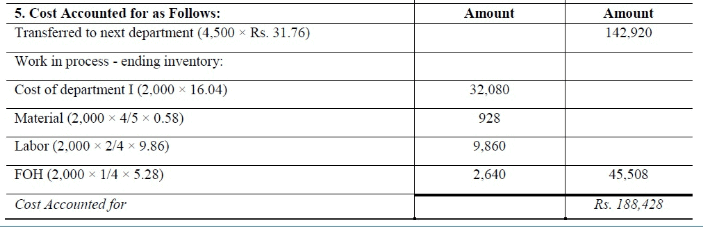Problem # 3:
Shah Manufacturing Company engaged in a continuous process, manufacturing a single product which is processed in three departments known as Cleaning, Milling and Finishing. There were no inventories at beginning of the March 2018.  Following data is available for preparing Cost of Production Report for three departments: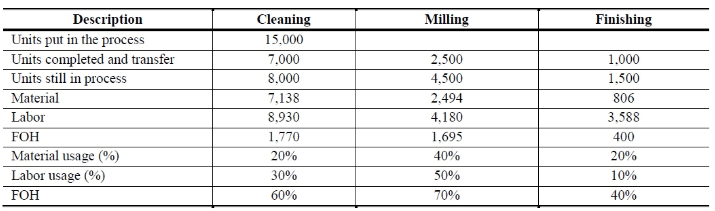Solution: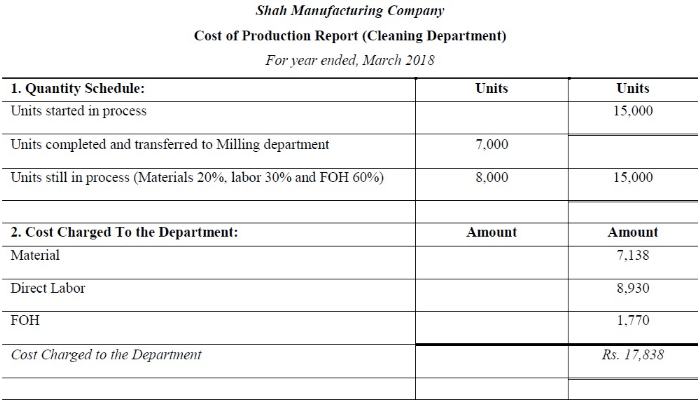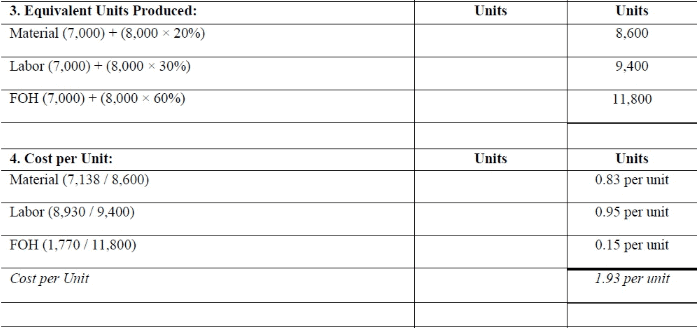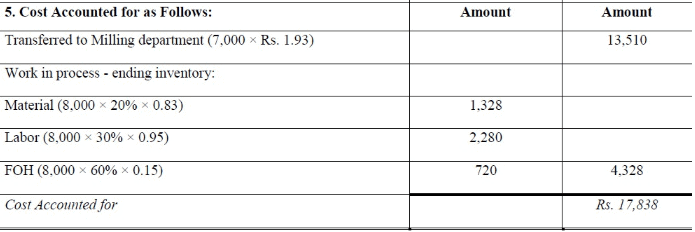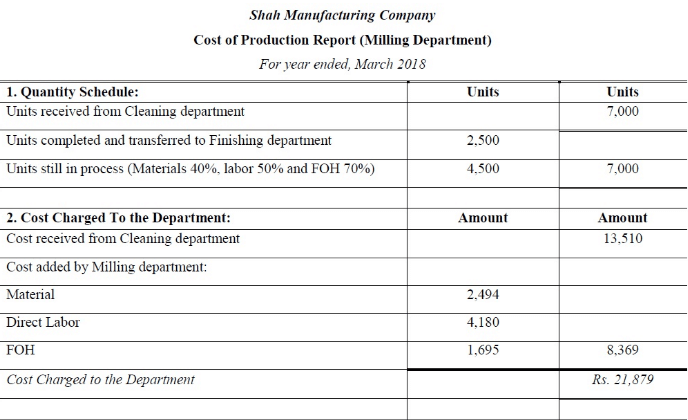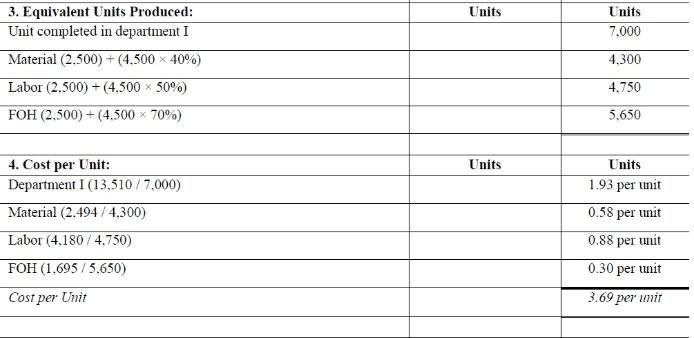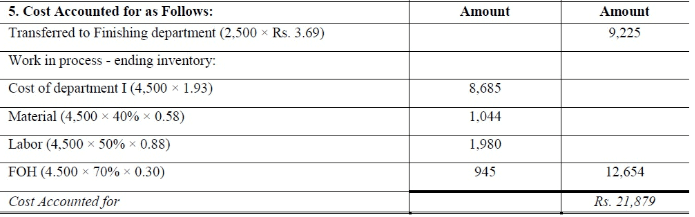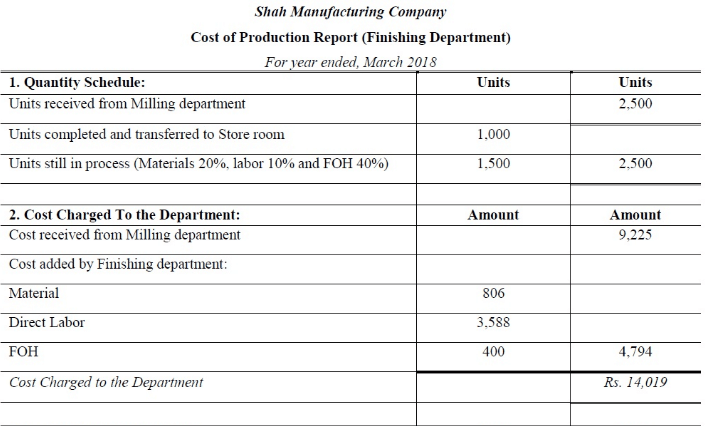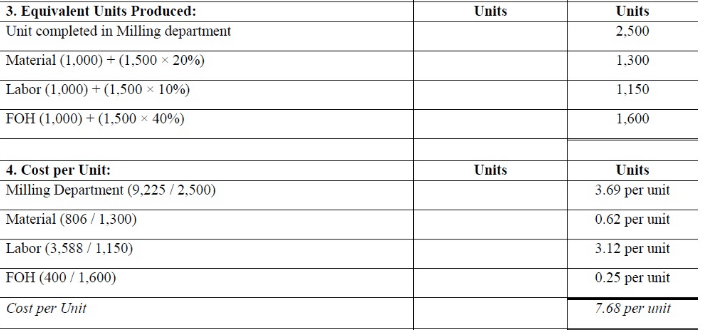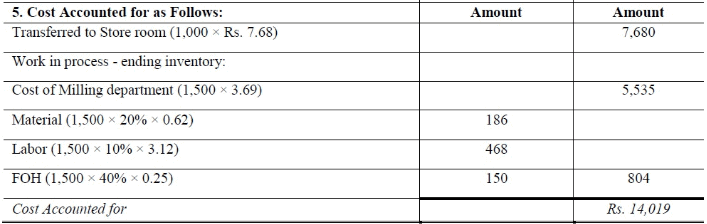Problem 4:
Tazeen Ltd. is manufacturing a single product which is being processed in three departments called as Molding, Painting and Finishing. There were no inventories at beginning of the March 2018.  Following data is available for preparing Cost of Production Report for three departments: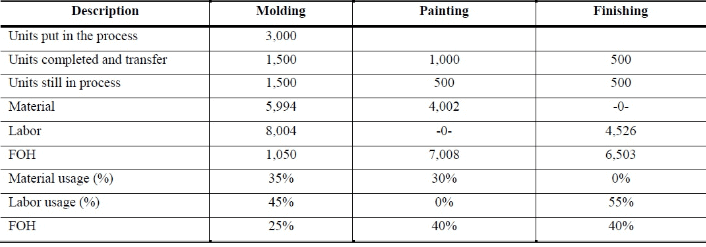Solution: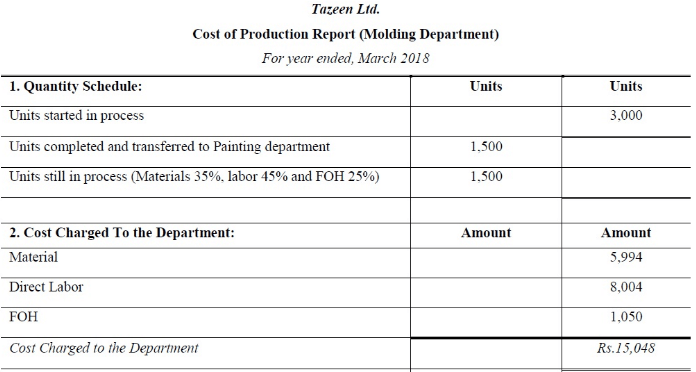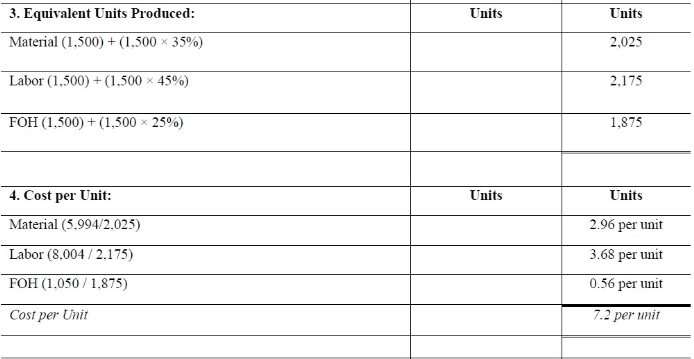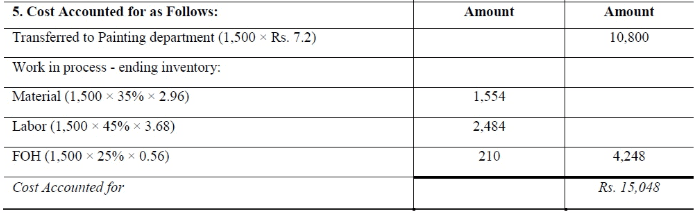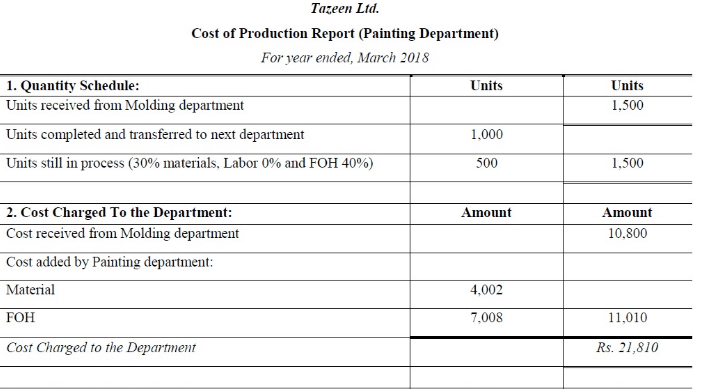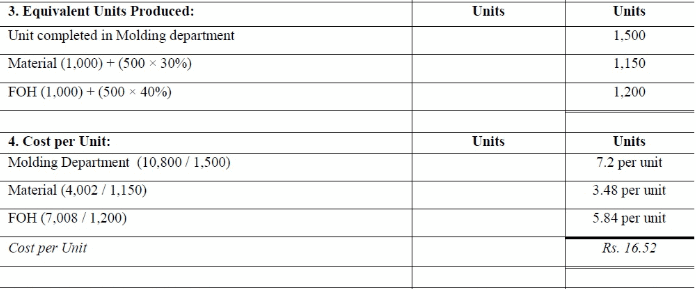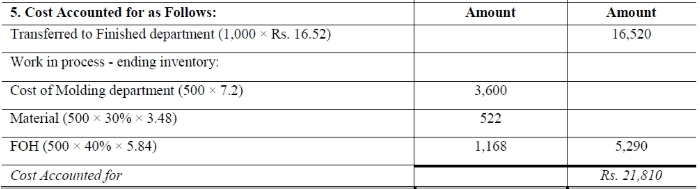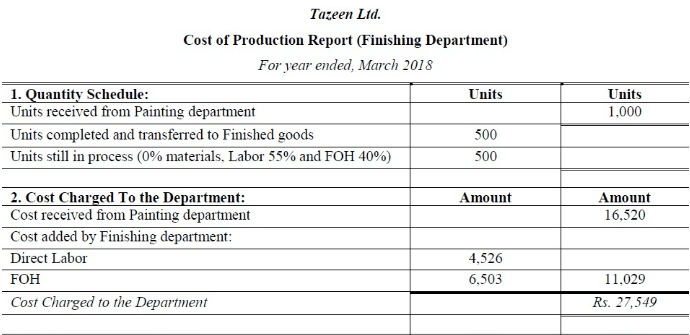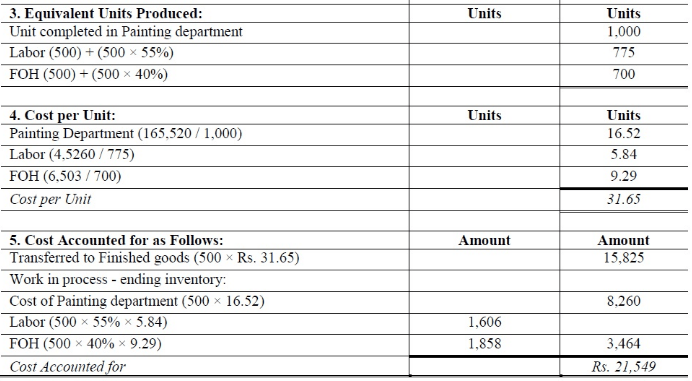Offer running on EduRev: Apply code STAYHOME200 to get INR 200 off on our premium plan EduRev Infinity!

## Cost Accounting

131 videos|139 docs

,

,

,

,

,

,

,

,

,

,

,

,

,

,

,

,

,

,

,

,

,

,

,

,

;